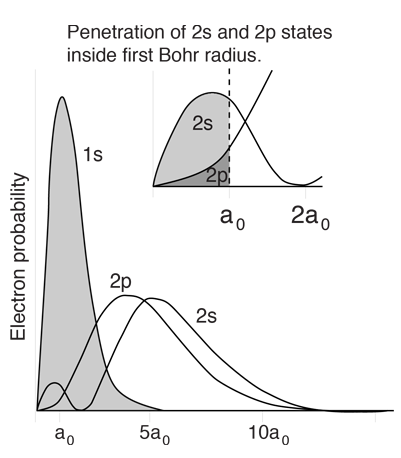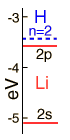# Lithium Energy LevelsThe lithium atom has a closed n=1 shell with two electrons and then one electron outside. Since the outer electron looks inward at just one net positive charge, it could be expected to have energy levels close to those of hydrogen. This is true for high angular momentum states as shown, but the s and p states fall well below the corresponding hydrogen energy levels.

The general layout here was taken from Rohlf but some discrepancies were found in the lower levels. The levels up through the 3d plus the 4p and 4d were rescaled to fit the data in the table of neutral lithium levels from NIST. Discrepancies may remain with the other levels. The ionization potential given by NIST was 5.395 eV.

 Why do levels vary with orbital quantum number? Details for 2s and 2p levels
 Electron energy level diagrams
Index

Rohlf Sec 9.5, Fig 9.9

 HyperPhysics***** Quantum Physics R Nave
Go Back

# Lithium Energy Levels:Orbital DependenceThe lithium 2s level is significantly lower than the 2p because of greater penetration past the shielding of the 1s electron. Both levels penetrate enough to be significantly lower than the n=2 hydrogen energy which they would have if the shielding were perfect.The 2s electron is lowered about 1.7 eV below the n=2 hydrogenic energy level of -3.4 eV which it would have if the shielding were perfect.
The illustration above uses the hydrogen wavefunctions, which are not exactly correct for lithium but can be used to obtain a qualitative understanding of the dependence of the electron energies on the orbital quantum number. If there were no shielding of the 2s electron, it would be exposed to the entire nuclear charge and have energy -30.6 eV. Modeling this situation by dividing the electron's time between perfect shielding and no shielding, the percentage of time inside the shielding is calculated to be about 6% for the 2s electron. The same model for the 2p electron suggests only about 0.5% of its time inside the shielding.
 Why do levels vary with orbital quantum number?
Index

 HyperPhysics***** Quantum Physics R Nave
Go Back